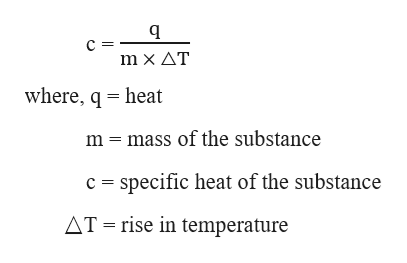# A 135 g sample of metal requires 2.50 kJ to change its temperature from 19.5 oC to 100.0 oC. What is the specific heat of this metal?

Question
2 views

A 135 g sample of metal requires 2.50 kJ to change its temperature from 19.5 oC to 100.0 oC. What is the specific heat of this metal?

check_circle

Step 1

Given,

Mass of the metal, m = 135 g

Heat required, q =2.50 kJ = 2500 J    (1 kJ = 1000 J)

Initial temperature, T1 = 19.5 oC

Final temperature, T2 = 100.0 oC

The specific heat required to rise the temperature of a substance is given as:help_outlineImage Transcriptionclosemx ΔT where, q heat mass of the substance m specific heat of the substance c AT rise in temperature fullscreen
Step 2

Here,

△T = T2 – T1 = 100.0 oC – 19.5 oC = 80.5 oC

Therefore, the specific he...

### Want to see the full answer?

See Solution

#### Want to see this answer and more?

Solutions are written by subject experts who are available 24/7. Questions are typically answered within 1 hour.*

See Solution
*Response times may vary by subject and question.
Tagged in

### Chemical Thermodynamics